Home > INT3 > Chapter 7 > Lesson 7.2.2 > Problem7-74

7-74.
1. Consider the transformations outlined below. Homework Help ✎

1. How is the graph of f(x) =transformed to obtain the graph of g(x) =– 3?

2. How is the graph of y = x2 transformed to obtain the graph of y = –(x – 1)2 + 20?

3. How is the graph of y =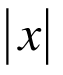transformed to obtain the graph of y = –3?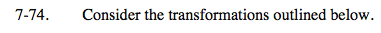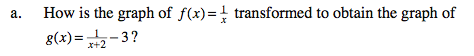There will be a vertical shift and a horizontal shift.

f is translated 2 units left and 3 units down to obtain g.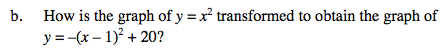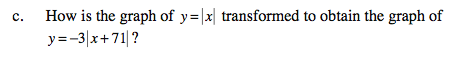To get the second graph, the first graph is vertically stretched by factor of 3, reflected
across the x-axis, and translated left 71 units.